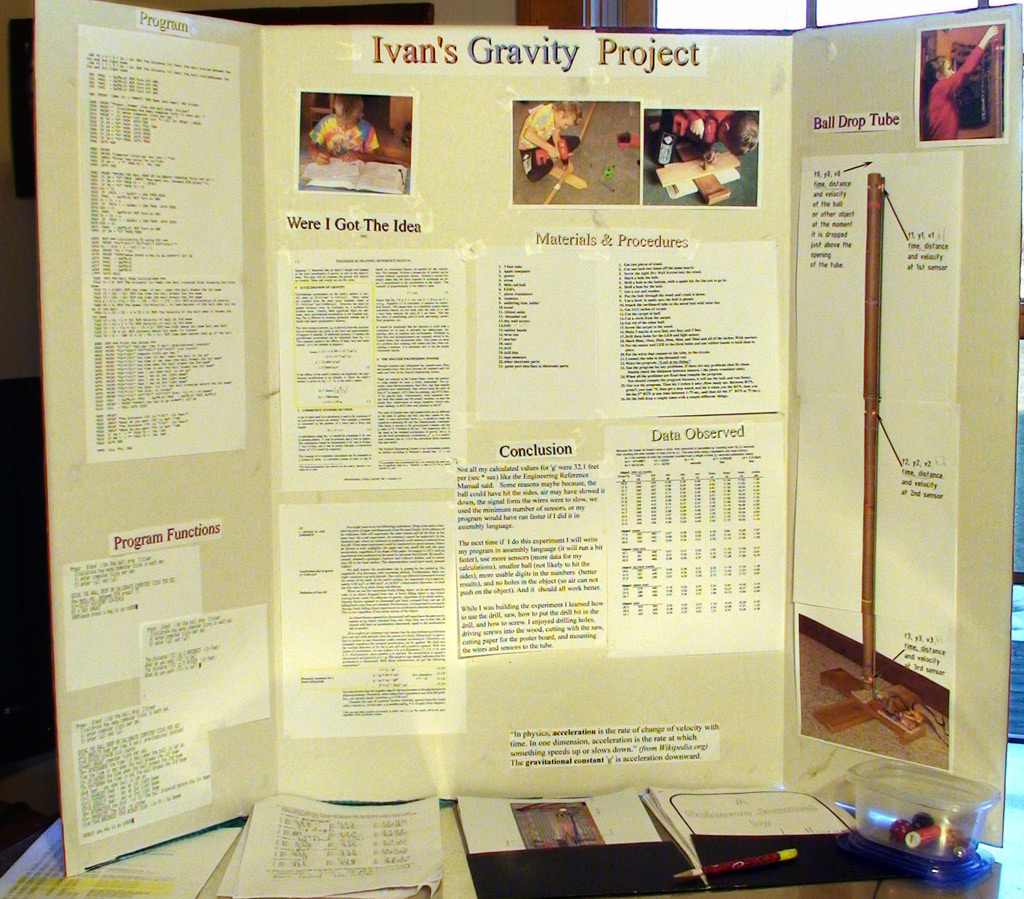### Calculating 'g' - Ivan's 2012 Science Fair Project

My February 2012 Science Fair project was based on some rummaging I did through my dad's EIT book. I have to admit I wasn't thrilled to do the project until my dad said I could use his power tools to build the ball drop tube. Building the tube stand, mounting the tube and helping my dad wire the sensors on the tube was fun. Writing the program turned out to be not as much fun. But, I got it done and it worked! (a couple times). My dad wired the breadboard where the tube sensors connected to the game port interface.

Purpose: The purpose of my Science Project was to calculate the gravitational constant 'g' by using a 5ft long cardboard tube, dropping a ball through the tube, then measuring the time it takes for the ball to drop 2ft and 5ft. In physics, acceleration is the rate of change of velocity with time. In one dimension, acceleration is the rate at which something speeds up or slows down. The earth's gravitational constant 'g' is acceleration downward.

Method: I used 3 light beams attached to the tube at y1=0ft, y2=2ft and y3=5ft. When a ball falls through the tube, it breaks the light beam. The Apple //e computer counts how long it takes from the first beam, to the second beam at 2ft, to the third beam at 5ft. The counts are converted to seconds. Using the 'kinematic equations for constant acceleration' found on-line or in a physics book, the computer can calculate 'g' because it measured the time from 0ft to 2ft to 5ft.

Equation: g = 2 * ( (5ft)/t3 - (2ft)/t2) ) / (t3 - t2)

More Data: The computer can also calculate the speed (a.k.a. velocity) the ball was falling at, when it passed each beam; and, how many inches above the top beam the person held the ball before it was let go.

Calibration: Because the Apple //e has no system clock [no way to get a timestamp], we had to calibrate a conversion factor 'ks'; ks is about 871 computer ticks per second. The same code used to calculate 'g' is used to calibrate 'ks'. That code counts 'ticks' between the time that the first, second and third light beams are broken. My program can calibrate 'ks' when a person presses BTN0 followed by BTN1, then BTN2; and, then enters how many seconds pass between button presses. I used a stopwatch and a 75 second interval between BTN0 and BTN2.

Data Observed: I dropped a lot of stuff down the tube: marbles, eraser, chap stick, shell, toy snail, etc. A lot of stuff hit the sides of the tube. A few marble drops did not. The Apple //e calculated 'g' to be anything between 17.5 to 32.1 ft / (sec ^ 2)

Conclusion: Not all the calculated values for 'g' were 32.1 because the stuff dropped down the tube either hit the sides or was slowed by air.

What I learned: While I was building the experiment I learned how to use the drill, saw, how to put the drill bit in the drill, and how to screw. I enjoyed drilling holes, driving screws into the wood, cutting with the saw, cutting paper for the poster board, and mounting the wires and sensors to the tube. I also learned that writing code that deals with real world I/O can be tricky.